### Home > PC > Chapter 2 > Lesson 2.3.3 > Problem2-101

2-101.
1. Given y =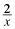for 4 ≤ x ≤ 6: Homework Help ✎

1. Divide the interval [4, 6] into 5 equal pieces. What is the width of each rectangle?

2. Find x0, x1, x2, x3, x4, and x5.

3. Use 5 rectangles to get upper and lower bounds for the area under the curve.

4. What minor change could you make to part (a) to make the upper and lower bounds closer together?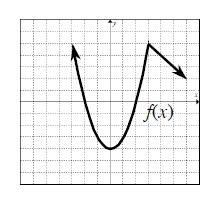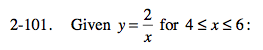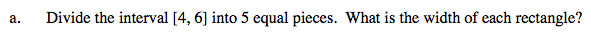$\frac{\text{interval length}}{\text{number of pieces}}$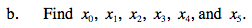x0 = 4
Now add the width to x0 to find x1. Continue the same for the last two.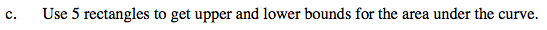Review homework problem 2-98 for the process.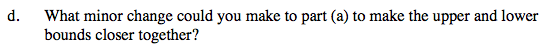Review homework problem 2-98.